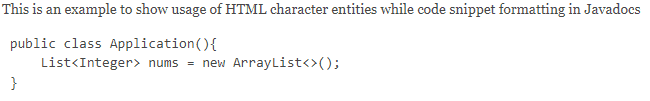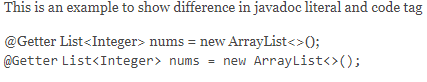## 2.简介

``````/**
* This is an example to show default behavior of code snippet formatting in Javadocs
*
* public class Application(){
*
* }
*
*/``````

### 3.1.使用<pre>标签

HTML提供了标记以指示预格式化文本。 它保留了所附文本的空格和换行符，从而保留代码段所需的缩进：

``````/**
* This is an example to show usage of HTML pre tag while code snippet formatting in Javadocs
*
* <pre>
* public class Application(){
*     List<Integer> nums = new ArrayList<>();
* }
*
* </pre>
*/``````### 3.2.使用HTML 性格 实体

``````/**
* This is an example to show usage of HTML character entities while code snippet formatting in Javadocs
*
* <pre>
* public class Application(){
*     List<Integer> nums = new ArrayList<>();
* }
*
* </pre>
*/``````### 3.3.使用 @code 标签

``````/**
* This is an example to show usage of javadoc code tag while code snippet formatting in Javadocs
*
* <pre>
*
* public class Application(){
*     {@code List<Integer> nums = new ArrayList<>(); }
* }
*
* </pre>
*/````````````/**
* This is an example to show issue faced while using annotations in Javadocs
*
* <pre>
*
* public class Application(){
*            @Getter
*     {@code List<Integer> nums = new ArrayList<>(); }
* }
*
* </pre>
*/````````````/**
* This is an example to show usage of javadoc code tag for handling '@' character
*
* <pre>
*
* public class Application(){
*     {@code @Getter}
*     {@code List<Integer> nums = new ArrayList<>(); }
* }
*
* </pre>
*/``````### 3.4.使用 @literal 标签

``````/**
* This is an example to show difference in javadoc literal and code tag
*
* <p>
*
* {@literal @Getter}
* {@literal List<Integer> nums = new ArrayList<>(); }
*
* <br />
* {@code @Getter}
* {@code List<Integer> nums = new ArrayList<>(); }
* </p>
*/``````### 3.5.格式化jQuery代码段

``````/**
* This is an example to illustrate a basic jQuery code snippet embedded in documentation comments
* <pre>
* {@code <script>}
*     console.log("Hello World!);
* })
* {@code </script>}
* </pre>
*/``````### 3.6.格式化HTML代码段

``````/**
* This is an example to illustrate an HTML code snippet embedded in documentation comments
* <pre>
* <html>
* <body>
* <h1>Hello World!</h1>
* </body>
* </html>
* </pre>
*
*/````````````/**
* This is an example to illustrate an HTML code snippet embedded in documentation comments
* <pre>{@code
* <html>
* <body>
* <h1>Hello World!</h1>
* </body>
* </html>}
* </pre>
*
*/``````## 4.结论

##### 热门推荐
•浏览(1,883)
•浏览(1,872)
•浏览(1,742)
•浏览(1,716)
•浏览(1,622)
•浏览(1,530)
•浏览(1,392)
•浏览(1,303)
•浏览(1,290)
•浏览(1,256)